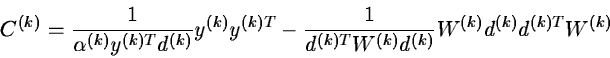Next: The Corrected Gauss-Newton No Up: Outline of the Available Previous: The Modified Gauss-Newton Method.

## The Quasi-Newton Method.

This is identical to the modified Gauss-Newton method, except in the way that the Hessian matrix is approximated.

This matrix is first initiated to zero. At each iteration, a new estimation of the Hessian is obtained by adding a rank one or two correction matrix to the last estimate such that H(k+1), the estimate of the Hessian matrix at the k+1th iteration, satisfies

(J(a(k+1))T J(a(k+1)) + H(k+1)) (x(k+1)-x(k)) = J(a(k+1)r(a(k+1)) - J(a(k)r(a(k))

The so-called BFGS updating formulas are applied in this algorithm

H(0) = 0     H(k+1) = H(k) + C(k)where

W(k)=J(a(k+1))T J(a(k+1))+H(k)

and

y(k)=J(a(k+1))r(a(k+1))-J(a(k))r(a(k))  ,

please see Gill, Murray and Pitfield (1972) for more details. After some iterations and around the optimum, H(k) converges to the Hessian.

This method requires the knowledge of the derivatives and, as the gradients are only computed once per iteration and consequently, the Hessian is more roughly approximated than with the modified Gauss-Newton method, this is better designed for a great number of parameters i.e. p > 10.Next: The Corrected Gauss-Newton No Up: Outline of the Available Previous: The Modified Gauss-Newton Method.
http://www.eso.org/midas/midas-support.html
1999-06-09